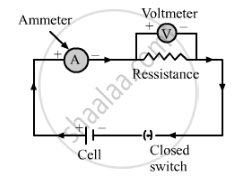# Draw the Labelled Diagram of an Electric Circuit Comprising of a Cell, a Resistance, an Ammeter, a Voltmeter and a Closed Switch (Or Closed Plug Key). Which of the Two Has a Large Resistance : an Ammeter Or a Voltmeter? - Science

Draw the labelled diagram of an electric circuit comprising of a cell, a resistance, an ammeter, a voltmeter and a closed switch (or closed plug key). Which of the two has a large resistance : an ammeter or a voltmeter?

#### Solution

Here is the diagram of an electric circuit comprising a cell, a resistance, an ammeter, a voltmeter and a closed switchA voltmeter has a high resistance so that it takes only a negligible amount of current from the circuit.

Concept: Electric Circuit
Is there an error in this question or solution?

#### APPEARS IN

Lakhmir Singh Class 10 Physics (Science)
Chapter 1 Electricity
Q 22.2